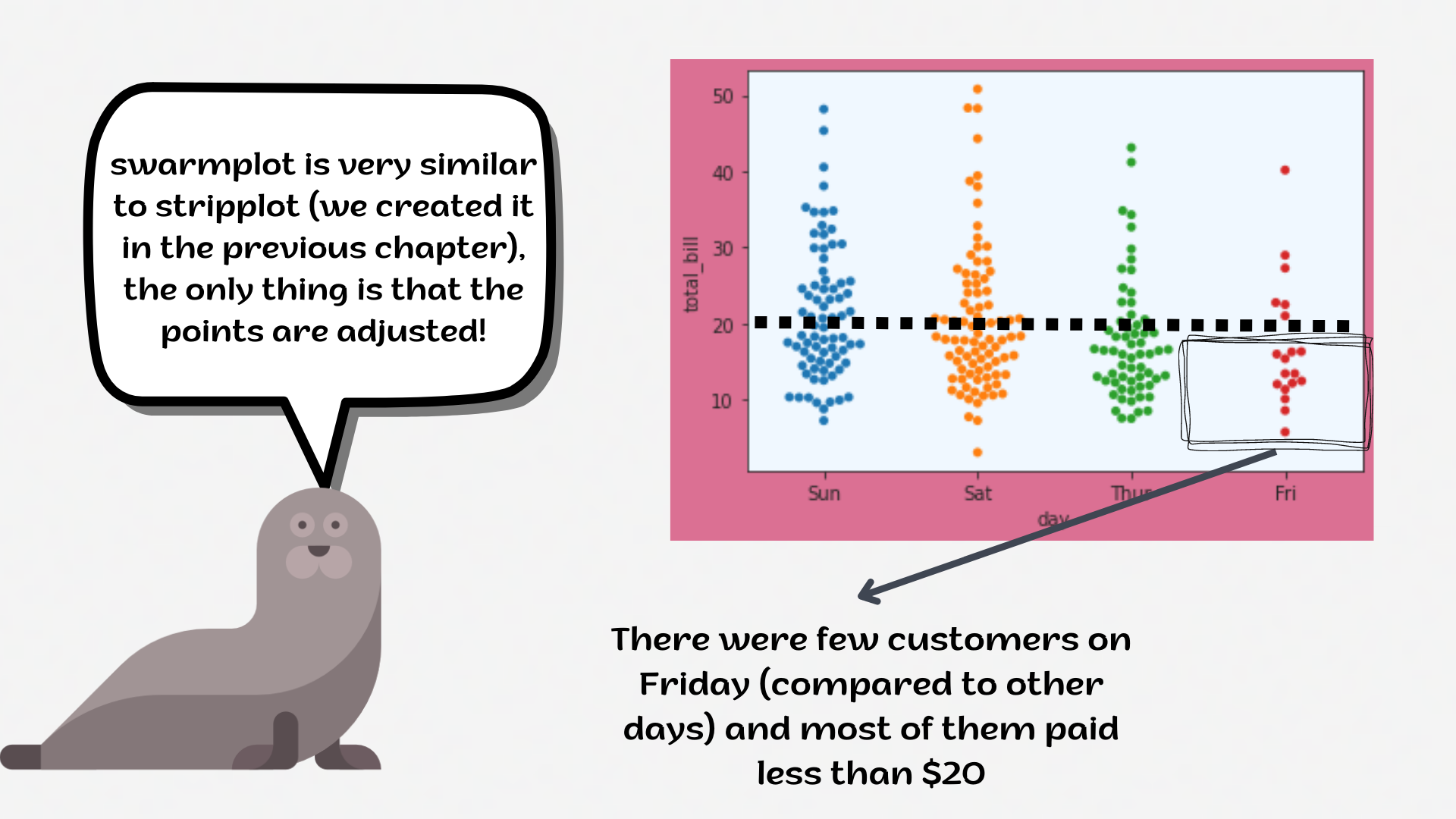Course Content

# Deep Dive into the seaborn Visualization

1. Light Start

2. Distributions of Data

3. Categorical Plot Types

4. Matrix Plots

5. Multi-Plot Grids

6. Regression Models

Deep Dive into the seaborn Visualization

##SwarmplotThe `swarmplot` is similar to `stripplot`. Only the points are adjusted so they won't overlap, as it helps to represent the distribution of values better.1. Set the `'whitegrid'` style with the `'seashell'` `axes.facecolor`.
2. Create the `swarmplot` using the `seaborn` library:
• `x` parameter equals the `'day'`;
• Set the `y` parameter equals the `'total_bill'`;
• Set the `hue` parameter equals the `'sex'`;
• Set the `linewidth` parameter equals `1`;
• Set the `size` parameter equals `2`;
• Set the `dodge` parameter equals the `True`;
• Set the `'rocket'` palette.

Everything was clear?

Section 3. Chapter 2